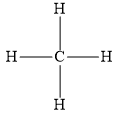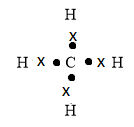Explain the bonding in methane molecules using electron dot structure.Verified
119.1k+ views
Hint: The chemical formula of methane molecule is ${\text{C}}{{\text{H}}_4}$. It contains four carbon-hydrogen sigma bonds.

The atomic numbers of hydrogen and carbon are 1 and 6 respectively. The electronic configurations of hydrogen and carbon are $1{s^1}$ and $1{s^2}2{s^2}2{p^2}$ respectively. Thus, the number of valence electrons for hydrogen and carbon are 1 and 4 respectively. To attain stability hydrogen should have 2 electrons in its valence shell. For this purpose, a hydrogen atom shares one electron with carbon atom, the carbon atom also shares one electron with this hydrogen atom. Thus, a carbon-hydrogen covalent bond is formed. To attain stability, the carbon atom needs eight electrons in its valence shell. For this purpose, the carbon atom shares 4 electrons with 4 hydrogen atoms to form 4 carbon-hydrogen covalent bonds.Note: Since the central carbon atom in methane molecule has 4 bonds and zero lone pairs of electrons, it will undergo $s{p^3}$ hybridization to form four degenerate $s{p^3}$ hybrid orbitals. These four degenerate $s{p^3}$ hybrid orbitals of carbon overlap with four 1s atomic orbitals of 4 hydrogen atoms to form four carbon-hydrogen covalent bonds. Methane molecules have tetrahedral geometry.